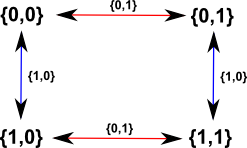# Maths - External Group Product

here the group elements of the resulting group are sets containing an element from each on the multiplicands.

### Example 1

Lets take the simplest example we can think of. Lets take the group Z2 which is the integers modulo two which gives an exclusive or table:

Cayley Table
Cayley Graph
0 1
0 0 1
1 1 0Lets combine two of these groups to give: Z2Z2

{g, h} × {g' , h' } = {g * g' , h o h' }

where:

• × is the operation of the combined algebra.
• * is the operation of the group G.
• o is the operation of the group H which may be, or may not be, the same as *.
Cayley Table
{0,0} {0,1} {1,0} {1,1}
{0,0} {0*0,0o0}={0,0} {0*0,0o1}={0,1} {0*1,0o0}={1,0} {0*1,0o1}={1,1}
{0,1} {0*0,1o0}={0,1} {0*0,1o1}={0,0} {0*1,1o0}={1,1} {0*1,1o1}={1,0}
{1,0} {1*0,0o0}={1,0} {1*0,0o1}={1,1} {1*1,0o0}={0,0} {1*1,0o1}={0,1}
{1,1} {1*0,1o0}={1,1} {1*0,1o1}={1,0} {1*1,1o0}={0,1} {1*1,1o1}={0,0}
Cayley GraphIs there any way we can modify this to generate the complex numbers?

Can we then go on to generate the quaternions and octonions?

• H = CC
• O = HH

Where I can, I have put links to Amazon for books that are relevant to the subject, click on the appropriate country flag to get more details of the book or to buy it from them.Contemporary Abstract Algebra written by Joseph Gallian - This investigates algebra (Groups, Rings, Fields) using an abstract (set theory) approach. It is very readable for such an abstract topic but the inclusion of 1 page histories of the great mathematicians and other background information.